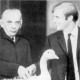# Gracious Living

Banach-Tarski part 1
November 21, 2010, 06:33
Filed under: Algebra, Math | Tags: , , , , , ,

Okay, here’s the moment you’ve been waiting for: the proof of the Banach-Tarski Paradox.  Here’s what the paradox says:

Theorem (Banach-Tarski).  There are a finite number of disjoint subsets of$\mathbb{R}^3$ whose union is the unit ball, and such that we can apply an isometry to each of them and wind up with disjoint sets whose union is a pair of unit balls.

Or “we can cut a unit ball up into a finite number of pieces, rearrange them, and put them back together to make two balls.”

The original statement was written in terms of equidecomposability.  Suppose a group$G$ acts on a set$X$.  Then$A,B\subset X$ are$G$equidecomposable if there is a partition of$A$ into sets$A_i$, of$B$ into sets$B_i$, and a list$g_i$ of elements of$G$, where in all cases,$i$ ranges from$1$ to the same finite number$k$, such that$g_i(A_i)=B_i$ for each$i$. We call a set paradoxical under the$G$-action if it can be partitioned into two proper subsets such that it’s equidecomposable with both. We’ll write$\sim$ or$\sim_G$ for equidecomposability, which is clearly an equivalence relation.

So basically what we’re trying to show is that the unit ball is$E(3)$-equidecomposable with two unit balls (where$E(3)=\mathbb{R}^3\rtimes SO(3)$ is the group of isometries of space). This makes the pair of unit balls$E(3)$-paradoxical.

Let’s start with a simple example. I claim that the unit circle in the plane is$E(2)$-equidecomposable with itself minus a point. To prove this, let$r\in E(2)$ be an “irrational rotation” — 1 radian, or$\sqrt{2}$ degrees, or some other rotation that isn’t expressible as a rational number of full rotations. In particular, as an element of$E(2)$,$r$ can’t have finite order. Let$p$ be a point in the circle. Then$p,rp,r^2p,r^3p,\dotsc$ must all be different points (since$r^k$ is a rotation, if it fixes one point, it must fix them all, which contradicts$r$ being of infinite order). If we apply$r$ to$\{p,rp,r^2p,\dotsc\}$, we get$\{rp,r^2p,r^3p,\dotsc\}$, which is the original set minus a point. Then just apply the identity to the rest of the circle, and the union of the two maps is a map from the circle to the circle minus a point, fitting the definition of equidecomposability above.

We’re actually going to use this later — in particular, it shows that any set in the plane or space that contains a circle is equidecomposable to itself minus a point.

Okay, now let’s get a little more abstract. Remember when we talked about free groups? It turns out that the free group on two generators,$F_2$, is paradoxical under its action on itself. Here’s why. Let$W(a)$ be the set of reduced words starting with the generator$a$, and similarly for$W(b),W(a^{-1}),W(b^{-1})$.  Then these four sets and the singleton set$\{e\}$ partition$F_2$.  But$aW(a^{-1})$ is the set of all words that <em>don’t</em> start with$a$, so$aW(a^{-1})\cup W(a)=F_2$.  Similarly,$bW(b^{-1})\cup W(b)=F_2$.  So this is a paradoxical decomposition of$F_2$.

Or, okay, it’s not, but it almost is, because I conveniently forgot about$e$ above.  But this doesn’t break everything — just add it to one of the other sets, say$W(a^{-1})$.  And since we want the sets to stay disjoint, let’s also add$a,a^2,a^3,\dotsc$ to$W(a^{-1})$.  Then$aW(a^{-1})$ is disjoint from$W(a)$,$bW(b^{-1})$ is disjoint from$W(b)$, and each pair partitions$F_2$.

But, see, we can apply this directly to the unit ball — because there’s an embedding of$F_2$ into$SO(3)$.  There are different ways of doing this, but the basic idea is to take two irrational rotations about different axes and show they satisfy no relations.  I like Terry Tao’s smooth proof for this part of the argument.  Let$a(x,y,z)=(\frac{3}{5}x+\frac{4}{5}y,\frac{-4}{5}x+\frac{3}{5}y,z),b(x,y,z)=(x,\frac{3}{5}y+\frac{4}{5}z,\frac{-4}{5}y+\frac{3}{5}z)$.  By writing down these guys in matrix form, you can check that they’re special orthogonal, and also that their inverses are given by just inverting the signs on the$\frac{4}{5}$s.  The trick now is to look at everything modulo 5.  See, if$a$ and$b$ have a nontrivial relation, then it translates to a word in$5a$ and$5b$ whose coefficients of$x,y,z$ are all divisible by$5$, so if we can show that one of these doesn’t exist, we’re done.  So we work over the finite field$\mathbb{F}_5$, which is$\mathbb{Z}_5$ additively and the multiplication is induced from that.  Mod 5,$5a(x,y,z)=(3x+4y,-4x+3y,0),5b(x,y,z)=(0,3y+4z,-4y+3z)$.  It’s pretty clear that the kernels of these maps are$2$-dimensional vector spaces mod 5: the kernel of$5a$, for instance, is $latez \langle (0,0,1),(3,4,0)\rangle$.  By a theorem of linear algebra, their images are thus$1$-dimensional.  As it turns out, the images are perpendicular, so there’s no nontrivial relation in$\mathbb{F}_5$.

Later today, I’ll bring this all together.  Sorry this was late!